# The SEQTEST Procedure

### Example 90.3 Testing an Effect with Early Stopping to AcceptThis example demonstrates a two-sided group sequential test that uses an error spending design with early stopping to accept the null hypothesis. The example is similar to Example 90.2 but with early stopping to accept.

A study is conducted to examine the effects of Age (years), Weight (kg), RunTime (time in minutes to run 1.5 miles), RunPulse (heart rate while running), and MaxPulse (maximum heart rate recorded while running) on Oxygen (oxygen intake rate, ml per kg body weight per minute). The primary interest is whether oxygen intake rate is associated with weight.

The hypothesis is tested using the following linear model: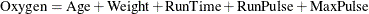The null hypothesis is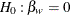, where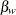is the regression parameter for the variable Weight. Suppose that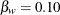is the reference improvement that should be detected at a 0.90 level. Then the maximum information
can be derived in the SEQDESIGN procedure.

Following the derivations in the section "Test for a Parameter in the Regression Model" in the chapter "The SEQDESIGN Procedure," the required sample size can be derived from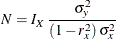where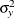is the variance of the response variable in the regression model,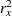is the proportion of variance of Weight explained by other covariates, and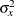is the variance of Weight.

Further suppose that from past experience,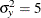,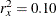, and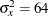. Then the required sample size can be derived using the SAMPLESIZE statement in the SEQDESIGN procedure.

The following statements invoke the SEQDESIGN procedure and request a three-stage group sequential design for normally distributed data to test the null hypothesis of a regression parameteragainst the alternative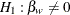:

ods graphics on;
proc seqdesign altref=0.10;
OBFErrorFunction: design method=errfuncgamma
stop=accept
nstages=3
info=cum(2 3 4);

samplesize model=reg( variance=5 xvariance=64 xrsquare=0.10);
ods output Boundary=Bnd_Fit;
run;
ods graphics off;


By default (or equivalently if you specify ALPHA=0.05 and BETA=0.10), the procedure uses a Type I error probability 0.05 and a Type II error probability 0.10. The ALTREF=0.10 option specifies a power of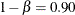at the alternative hypothesis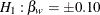. The INFO=CUM(2 3 4) option specifies that the study perform the first interim analysis with information proportion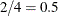—that is, after half of the total observations are collected.

The ODS OUTPUT statement with the BOUNDARY=BND_FIT option creates an output data set named BND_FIT which contains the resulting boundary information for the subsequent sequential tests.

The "Design Information" table in Output 90.3.1 displays design specifications and derived statistics. Since the alternative reference is specified, the maximum information is derived.

Output 90.3.1: Error Spending Design Information

The SEQDESIGN Procedure
Design: OBFErrorFunction

Design Information
Statistic Distribution Normal
Boundary Scale Standardized Z
Alternative Hypothesis Two-Sided
Early Stop Accept Null
Method Error Spending
Boundary Key Both
Alternative Reference 0.1
Number of Stages 3
Alpha 0.05
Beta 0.1
Power 0.9
Max Information (Percent of Fixed Sample) 103.9245
Max Information 1091.972
Null Ref ASN (Percent of Fixed Sample) 75.00521
Alt Ref ASN (Percent of Fixed Sample) 101.8099

The "Boundary Information" table in Output 90.3.2 displays information level, alternative reference, and boundary values at each stage.

Output 90.3.2: Boundary Information

Boundary Information (Standardized Z Scale)
Null Reference = 0
_Stage_   Alternative Boundary Values
Information Level Reference Lower Upper
Proportion Actual N Lower Upper Beta Beta
1 0.5000 545.9862 47.39463 -2.33663 2.33663 -0.44937 0.44937
2 0.7500 818.9792 71.09195 -2.86178 2.86178 -1.13583 1.13583
3 1.0000 1091.972 94.78926 -3.30450 3.30450 -1.91428 1.91428

With ODS Graphics enabled, a detailed boundary plot with the rejection and acceptance regions is displayed, as shown in Output 90.3.3. The boundary plot also displays the information level and critical value for the corresponding fixed-sample design.

Output 90.3.3: Boundary Plot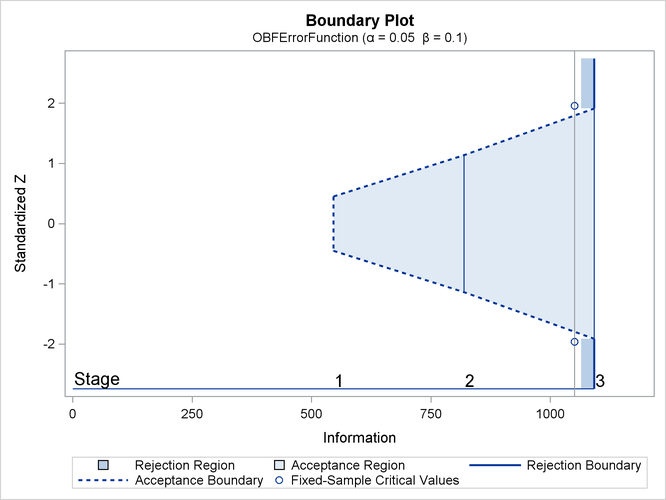With the MODEL=REG option in the SAMPLESIZE statement, the "Sample Size Summary" table in Output 90.3.4 displays the parameters for the sample size computation.

Output 90.3.4: Required Sample Size Summary

Sample Size Summary
Test Reg Parameter
Parameter 0.1
Variance 5
X Variance 64
R Square (X) 0.1
Max Sample Size 94.78926
Expected Sample Size (Null Ref) 68.41207
Expected Sample Size (Alt Ref) 92.86057

The "Sample Sizes" table in Output 90.3.5 displays the required sample sizes for the group sequential clinical trial.

Output 90.3.5: Required Sample Sizes

Sample Sizes (N)
Z Test for Regression Parameter
_Stage_ Fractional N Ceiling N
N Information N Information
1 47.39 546.0 48 553.0
2 71.09 819.0 72 829.4
3 94.79 1092.0 95 1094.4

Thus, 48, 72, and 95 individuals are needed in stages 1, 2, and 3, respectively. Since the sample sizes are derived from estimated values of,, and, the actual information levels might not achieve the target information levels. Thus, instead of specifying sample sizes in the protocol, you can specify the maximum information levels. Then if an actual information level is much less than the target level, you can increase the sample sizes for the remaining stages to achieve the desired information levels and power.

Suppose that 48 individuals are available at stage 1. Output 90.3.6 lists the first 10 observations of the trial data.

Output 90.3.6: Clinical Trial Data

 First 10 Obs in the Trial Data

Obs Oxygen Age Weight RunTime RunPulse MaxPulse
1 54.5521 44 87.7676 11.6949 178.435 181.607
2 52.2821 40 75.4853 9.8872 184.433 183.667
3 62.1871 44 89.0638 8.7950 155.540 167.108
4 65.3269 42 67.7310 8.4577 162.926 173.877
5 59.9809 37 93.1902 9.3228 179.033 180.144
6 52.5588 47 75.9044 12.0385 177.753 175.033
7 51.7838 40 73.5422 11.6607 175.838 178.140
8 57.0024 43 81.2861 11.2219 160.963 171.770
9 48.0775 44 85.2290 13.1789 173.722 176.548
10 68.3357 38 80.2490 8.5066 171.824 184.011

The following statements use the REG procedure to estimate the slopeand its associated standard error at stage 1:

proc reg data=Fit_1;
model Oxygen=Age Weight RunTime RunPulse MaxPulse;
ods output ParameterEstimates=Parms_Fit1;
run;


The following statements create and display (in Output 90.3.7) the input data set that contains slopeand its associated standard error for the SEQTEST procedure:

data Parms_Fit1;
set Parms_Fit1;
if Variable='Weight';
_Scale_='MLE';
_Stage_= 1;
keep _Scale_ _Stage_ Variable Estimate StdErr;
run;

proc print data=Parms_Fit1;
title 'Statistics Computed at Stage 1';
run;


Output 90.3.7: Statistics Computed at Stage 1

 Statistics Computed at Stage 1

Obs Variable Estimate StdErr _Scale_ _Stage_
1 Weight 0.04660 0.04308 MLE 1

The following statements invoke the SEQTEST procedure to test for early stopping at stage 1:

ods graphics on;
proc seqtest Boundary=Bnd_Fit
Parms(testvar=Weight)=Parms_Fit1
infoadj=none
errspendadj=errfuncgamma
stopprob
order=lr
;
ods output Test=Test_Fit1;
run;
ods graphics off;


The BOUNDARY= option specifies the input data set that provides the boundary information for the trial at stage 1, which was generated in the SEQDESIGN procedure. The PARMS=PARMS_FIT1 option specifies the input data set PARMS_FIT1 that contains the test statistic and its associated standard error at stage 1, and the TESTVAR=WEIGHT option identifies the test variable WEIGHT in the data set. The INFOADJ=NONE option maintains the information level for stage 2 at the value provided in the BOUNDARY= data set.

The ORDER=LR option uses the LR ordering to derive the p-value, the unbiased median estimate, and the confidence limits for the regression slope estimate. The ERRSPENDADJ=ERRFUNCGAMMA option adjusts the boundaries with the updated error spending values generated from a gamma cumulative error spending function.

The ODS OUTPUT statement with the TEST=TEST_FIT1 option creates an output data set named TEST_FIT1 which contains the updated boundary information for the test at stage 1. The data set also provides the boundary information that is needed for the group sequential test at the next stage.

The "Design Information" table in Output 90.3.8 displays the design specifications. By default (or equivalently if you specify BOUNDARYKEY=ALPHA), the boundary values are modified for the new information levels to maintain the Type Ilevel. The maximum information remains the same as in the BOUNDARY= data set, but the derived Type II error probabilityand power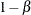are different because of the new information level.

Output 90.3.8: Design Information

The SEQTEST Procedure

Design Information
BOUNDARY Data Set WORK.BND_FIT
Data Set WORK.PARMS_FIT1
Statistic Distribution Normal
Boundary Scale Standardized Z
Alternative Hypothesis Two-Sided
Early Stop Accept Null
Number of Stages 3
Alpha 0.05
Beta 0.10007
Power 0.89993
Max Information (Percent of Fixed Sample) 103.9498
Max Information 1091.97232
Null Ref ASN (Percent of Fixed Sample) 75.15846
Alt Ref ASN (Percent of Fixed Sample) 101.8296

With the STOPPROB option, the "Expected Cumulative Stopping Probabilities" table in Output 90.3.9 displays the expected stopping stage and the cumulative stopping probability of accepting the null hypothesis at each stage under various hypothetical references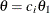, where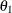is the alternative reference and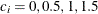by default. You can specify other values for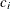with the CREF= option.

Output 90.3.9: Stopping Probabilities

Expected Cumulative Stopping Probabilities
Reference = CRef * (Alt Reference)
CRef Expected
Stopping Stage
Source Stopping Probabilities
Stage_1 Stage_2 Stage_3
0.0000 1.895 Accept Null 0.33304 0.76607 0.95000
0.5000 2.409 Accept Null 0.17680 0.40947 0.62828
1.0000 2.918 Accept Null 0.02636 0.05453 0.10007
1.5000 2.997 Accept Null 0.00109 0.00166 0.00242

The "Test Information" table in Output 90.3.10 displays the boundary values for the test statistic. By default (or equivalently if you specify BOUNDARYSCALE=STDZ), these statistics are displayed with the standardized Z scale. The information level at stage 1 is derived from the standard error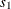in the PARMS= data set,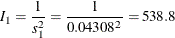Output 90.3.10: Sequential Tests

Test Information (Standardized Z Scale)
Null Reference = 0
_Stage_   Alternative Boundary Values Test
Information Level Reference Lower Upper Weight
Proportion Actual Lower Upper Beta Beta Estimate Action
1 0.4934 538.7887 -2.32118 2.32118 -0.43033 0.43033 1.08174 Continue
2 0.7500 818.9792 -2.86178 2.86178 -1.13623 1.13623 .
3 1.0000 1091.972 -3.30450 3.30450 -1.91431 1.91431 .

At stage 1, the standardized Z statistic 1.08174 is greater than the upperboundary 0.43033, so the trial continues to the next stage.

With ODS Graphics enabled, a boundary plot with test statistics is displayed, as shown in Output 90.3.11. As expected, the test statistic is in the continuation region.

Output 90.3.11: Sequential Test Plot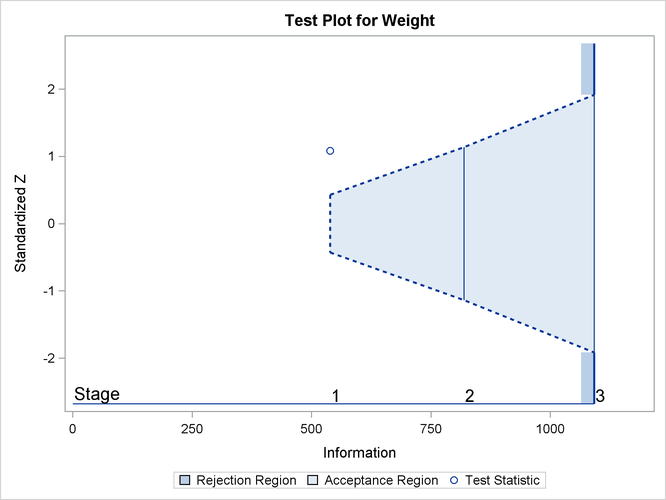The following statements use the REG procedure to estimate the slopeand its associated standard error at stage 2:

proc reg data=Fit_2;
model Oxygen=Age Weight RunTime RunPulse MaxPulse;
ods output ParameterEstimates=Parms_Fit2;
run;


Note that the data set Fit_2 contains both the data from stage 1 and the data from stage 2,

The following statements create and display (in Output 90.3.12) the input data set that contains slopeand its associated standard error at stage 2 for the SEQTEST procedure:

data Parms_Fit2;
set Parms_Fit2;
if Variable='Weight';
_Scale_='MLE';
_Stage_= 2;
keep _Scale_ _Stage_ Variable Estimate StdErr;
run;

proc print data=Parms_Fit2;
title 'Statistics Computed at Stage 2';
run;


Output 90.3.12: Statistics Computed at Stage 2

 Statistics Computed at Stage 2

Obs Variable Estimate StdErr _Scale_ _Stage_
1 Weight 0.02925 0.03490 MLE 2

The following statements invoke the SEQTEST procedure to test for early stopping at stage 2:

ods graphics on;
proc seqtest Boundary=Test_Fit1
Parms(testvar=Weight)=Parms_Fit2
errspendadj=errfuncgamma
order=lr
pss
plots=(asn power)
;
ods output Test=Test_Fit2;
run;
ods graphics off;


The BOUNDARY= option specifies the input data set that provides the boundary information for the trial at stage 2, which was generated by the SEQTEST procedure at the previous stage. The PARMS= option specifies the input data set that contains the test statistic and its associated standard error at stage 2, and the TESTVAR= option identifies the test variable in the data set.

Since the data set PARMS_FIT2 does not contain the test information at stage 1, the information level at stage 1 in the TEST_FIT1 data set is used to generate boundary values for the test.

The ORDER=LR option uses the LR ordering to derive the p-value, unbiased median estimate, and confidence limits for the regression slope estimate.

The ODS OUTPUT statement with the TEST=TEST_FIT2 option creates an output data set named TEST_FIT2 which contains the updated boundary information for the test at stage 2. The data set also provides the boundary information that is needed for the group sequential test at the next stage.

The "Design Information" table in Output 90.3.13 displays design specifications. By default (or equivalently if you specify BOUNDARYKEY=ALPHA), the boundary values are modified for the new information levels to maintain the Type Ilevel.

Output 90.3.13: Design Information

The SEQTEST Procedure

Design Information
BOUNDARY Data Set WORK.TEST_FIT1
Data Set WORK.PARMS_FIT2
Statistic Distribution Normal
Boundary Scale Standardized Z
Alternative Hypothesis Two-Sided
Early Stop Accept Null
Number of Stages 3
Alpha 0.05
Beta 0.10009
Power 0.89991
Max Information (Percent of Fixed Sample) 103.9566
Max Information 1091.97232
Null Ref ASN (Percent of Fixed Sample) 75.18254
Alt Ref ASN (Percent of Fixed Sample) 101.8349

The derived Type II error probabilityand powerare different because of the new information levels.

With the PSS option, the "Power and Expected Sample Sizes" table in Output 90.3.14 displays powers and expected mean sample sizes under various hypothetical references, whereis the alternative reference andare the default values in the CREF= option.

Output 90.3.14: Power and Expected Sample Size Information

Powers and Expected Sample Sizes
Reference = CRef * (Alt Reference)
CRef Power Sample Size
Percent
Fixed-Sample
0.0000 0.02500 75.1825
0.5000 0.37154 88.5975
1.0000 0.89991 101.8349
1.5000 0.99758 103.8843

With the PLOTS=ASN option, the procedure displays a plot of expected sample sizes under various hypothetical references, as shown in Output 90.3.15. By default, expected sample sizes under the hypotheses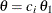,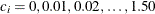, are displayed, whereis the alternative reference.

Output 90.3.15: ASN Plot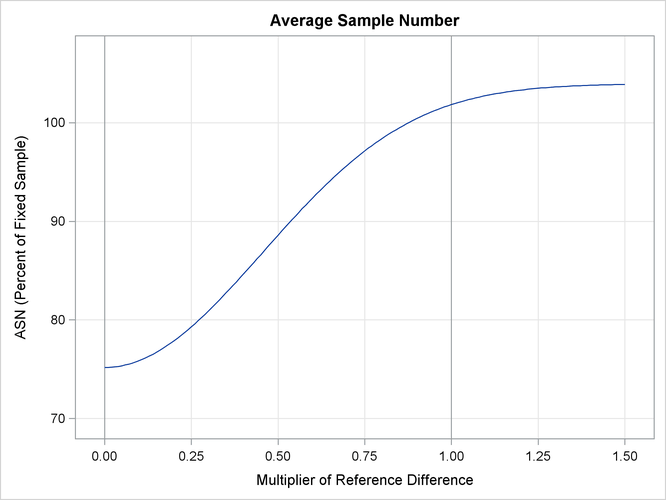With the PLOTS=POWER option, the procedure displays a plot of the power curves under various hypothetical references for all designs simultaneously, as shown in Output 90.3.16. By default, powers under hypothetical referencesare displayed, whereby default. You can specifyvalues with the CREF= option. Thevalues are displayed on the horizontal axis.

Output 90.3.16: Power Plot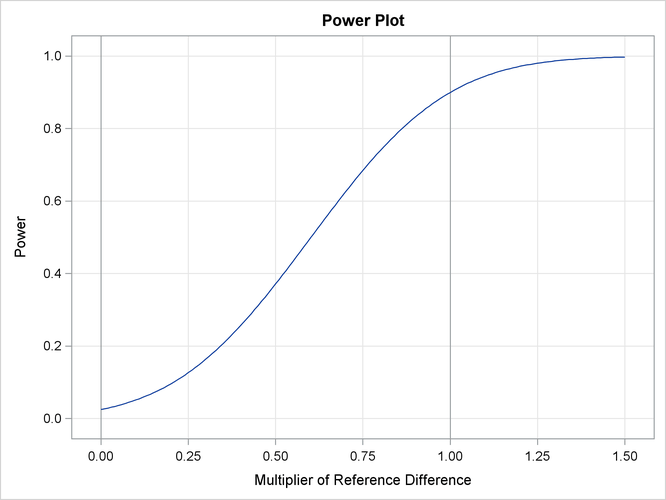Under the null hypothesis,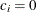, the power is 0.025, which is the upper Type I error probability. Under the alternative hypothesis,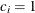, the power is 0.89991, which is one minus the Type II error probability, as displayed in the "Design Information" table in Output 90.3.13.

The "Test Information" table in Output 90.3.17 displays the boundary values for the test statistic with the default standardized Z scale. At stage 2, the standardized slope estimate 0.83805 is between the lower and upperboundary values. The trial stops to accept the null hypothesis that the variable Weight has no effect on the oxygen intake rate after adjusting for other covariates.

Output 90.3.17: Sequential Tests

Test Information (Standardized Z Scale)
Null Reference = 0
_Stage_   Alternative Boundary Values Test
Information Level Reference Lower Upper Weight
Proportion Actual Lower Upper Beta Beta Estimate Action
1 0.4934 538.7887 -2.32118 2.32118 -0.43033 0.43033 1.08174 Continue
2 0.7517 820.8509 -2.86505 2.86505 -1.14239 1.14239 0.83805 Accept Null
3 1.0000 1091.972 -3.30450 3.30450 -1.91408 1.91408 .

Since the data set PARMS_FIT2 contains the test information only at stage 2, the information level at stage 1 in the TEST_FIT1 data set is used to generate boundary values for the test.

With ODS Graphics enabled, a boundary plot with test statistics is displayed, as shown in Output 90.3.18. As expected, the test statistic is in the acceptance region between the lower and upperboundaries at the final stage.

Output 90.3.18: Sequential Test Plot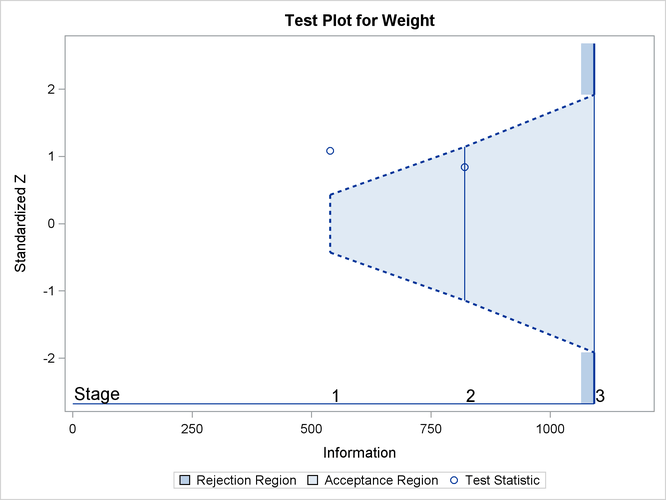After a trial is stopped, the "Parameter Estimates" table in Output 90.3.19 displays the stopping stage, parameter estimate, unbiased median estimate, confidence limits, and the p-value under the null hypothesis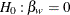. As expected, the p-value 0.3056 is not significant at the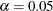level, and the confidence interval does contain the value zero. The p-value, unbiased median estimate, and confidence limits depend on the ordering of the sample space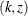, where k is the stage number and z is the standardized Z statistic. With the specified LR ordering, the p-values are computed with the orderingif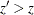. See the section Available Sample Space Orderings in a Sequential Test for a detailed description of the LR ordering.

Output 90.3.19: Parameter Estimates

Parameter Estimates
LR Ordering
Parameter Stopping
Stage
MLE p-Value for
H0:Parm=0
Median
Estimate
95% Confidence Limits
Weight 2 0.029251 0.3056 0.037080 -0.03368 0.10532